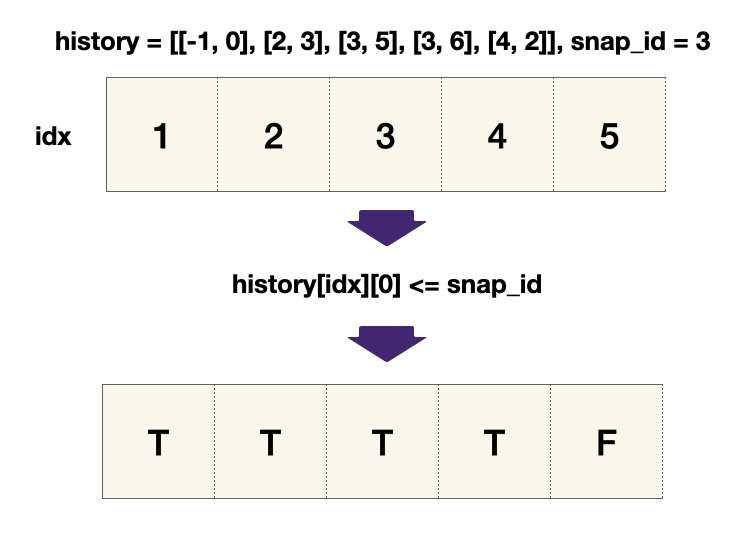# LeetCode Snapshot Array Solution

Implement a SnapshotArray that supports the following interface:

• `SnapshotArray(int length)` initializes an array-like data structure with the given length. Initially, each element equals 0.
• `void set(index, val)` sets the element at the given `index` to be equal to `val`.
• `int snap()` takes a snapshot of the array and returns the `snap_id`: the total number of times we called `snap()` minus `1`.
• `int get(index, snap_id)` returns the value at the given `index`, at the time we took the snapshot with the given `snap_id`

Example 1:

Input: ["SnapshotArray","set","snap","set","get"]

[,[0,5],[],[0,6],[0,0]]

Output: [null,null,0,null,5]

Explanation:

``````1SnapshotArray snapshotArr = new SnapshotArray(3); // set the length to be 3
2
3snapshotArr.set(0,5);  // Set array = 5
4
5snapshotArr.snap();  // Take a snapshot, return snap_id = 0
6
7snapshotArr.set(0,6);
8
9snapshotArr.get(0,0);  // Get the value of array with snap_id = 0, return 5``````

Constraints:

• `1 <= length <= 5 * 104`
• `0 <= index < length`
• `0 <= val <= 109`
• `0 <= snap_id <` (the total number of times we call `snap()`)
• At most `5 * 104` calls will be made to `set`, `snap`, and `get`.

## Solution

We wish to find the `pos` for the most recent value at the time we took the snapshot with the given `snap_id`, we are trying to find the rightmost index in `history=histories[i]` such that the `snap_id` at `history[pos]` is less or equal to the target `snap_id` (`a[i] <= snap_id`). This means that the feasible function is `a[i] <= snap_id`, whenever this is true, we must check the positions on its right to find the rightmost position that makes this condition hold.#### Implementation

``````1class SnapshotArray(object):
2
3def __init__(self, n):
4    # set up histories so that each index has its own history
5    self.histories = [[[-1, 0]] for _ in range(n)]
6    self.snap_id = 0
7
8def set(self, index, val):
9    self.histories[index].append([self.snap_id, val])
10
11def snap(self):
12    self.snap_id += 1
13    return self.snap_id - 1
14
15def get(self, index, snap_id):
16    left, right, pos = 0, len(self.histories[index])-1, -1
17    while left <= right:
18        mid = (left+right) // 2
19        if self.histories[index][mid] <= snap_id:
20            left = mid + 1
21            pos = mid
22        else:
23            right = mid - 1
24    return self.histories[index][pos]``````